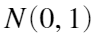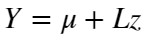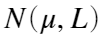## n-dimensional correlated Brownian motions

Version 1.0.1 (3.09 KB) by
Generate a matrix of increments from a multidimensional Brownian motion with a given vector of means and a Variance-Covariance matrix.

Updated 19 Sep 2022

From GitHub

Functions generate a matrix of increments from a multidimensional Brownian motion with a given vector of means and a Variance-Covariance matrix.
Problem
Offten when using for example multifactor models, the model requires correlated sources of noise. A popular choice is to use a multidimensional Brownian motion.
Solution
The proposed algorithm uses the fact that increments of a BM are normaly distributed as well as the fact that assuming n independent BM's whose increments are generated from a standard normal distribution (denoted, a derived proceshas its increments distributed aswhere mu is the vector of desired means and L is the square root of the desired Variance-Covariance matrix (denoted E).
Inputs
• Vecor of means for each BM `mu`
• Variance-Covariance matrix whose diagonal elements describe the volatility of each BM and the off-diagonal elements describe the covariance `E`
• Number of samples needed `sampleSize`
Output
• Matrix of samples where each column represents a BM and each row a new increment
Getting started
The user is interested in generating samples from 2 Brownian motions with a correlation of 0.8. Additionaly, the first BM has a mean of 1 and a variance of 1.5. The second BM has a mean of 0 and a variance of 2. The user is interested in 480 samples.
Example of use
mu = [1; 0];
VarCovar = [1.5, 0.8; 0.8, 2];
sampleSize = 480;
CorBrownian(mu, VarCovar, sampleSize)

### Cite As

Gregor Fabjan (2023). n-dimensional correlated Brownian motions (https://github.com/qnity/correlated_brownian_motion_matlab), GitHub. Retrieved .

##### MATLAB Release Compatibility
Created with R2021b
Compatible with any release
##### Platform Compatibility
Windows macOS Linux

### Community Treasure Hunt

Find the treasures in MATLAB Central and discover how the community can help you!

Start Hunting!